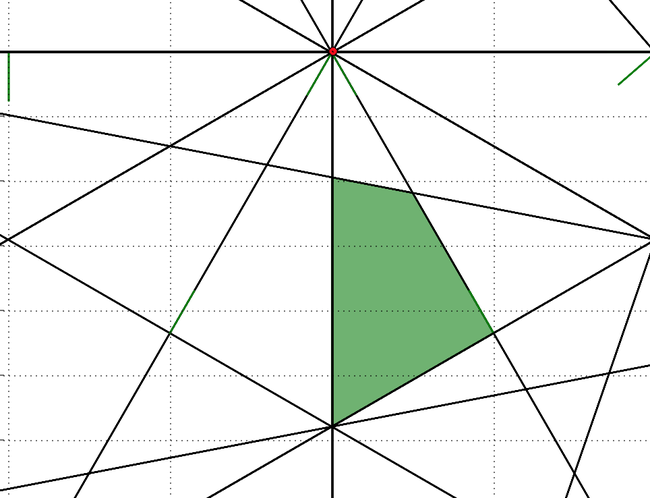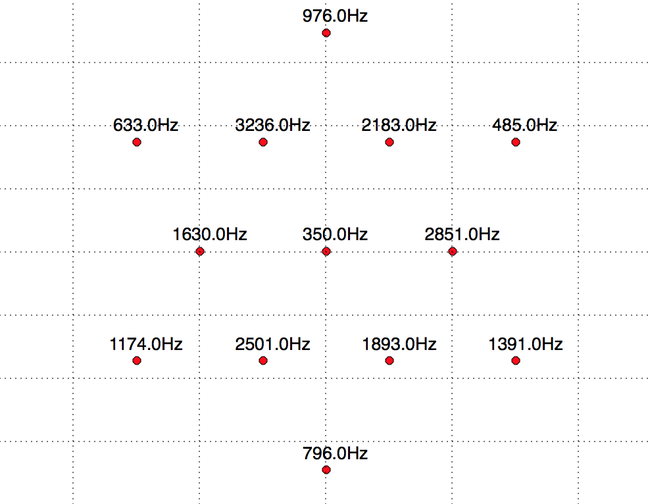# The Mighty Kalman Filter

A Kalman Filter is an algorithm for estimating the state of a system from a series of noisy measurements. That’s what I was told, and it confused me. It also doesn’t really do the Kalman filter justice. If we have a bunch of noisy measurements of something why don’t we just take the mean and be done with it? Well the impressive part is estimating the state of a system. This is something that is changing. Let’s says we have a state. This is usually a collection of variables that completely describe where something is and what it’s doing. For example if we are considering a cricket ball hit by a batsman and flying through the air towards the boundary it has a state described by its position which could be three numbers (`x`, `y`, `z`) and its velocity again (`vx`, `vy`, `vz`). In addition the cricket ball is under the effect of gravity so there are forces influencing some of the state. We also assume...

# Implementing a Star Camera

The orbit of a satellite can be computed from it’s location and velocity. What is more challenging is accurately determining its orientation. This is really important because satellites normally need to orient themselves to maximize the sunlight on their solar arrays and also point camera and other sensors in specific directions. There isn’t a lot to navigate with in space except the billions and billions of stars which is what a star camera does.

I thought it would be fun to try and implement a star camera. Let’s say we are given a picture of the night sky and need to determine which direction the camera is pointing in. To do this we need to compute a `direction` vector and also an `up` vector orthogonal to it so we know what the orientation of the camera is. For example, here is an image taken of Sirius, the brightest star in the sky. The un-cropped image in `1000` pixels square and the...

# Location Estimation - Part 2

In the previous article we attempted to solve this location problem. We used the power of each of a number of signals to roughly estimate our location. We managed to narrow it down to a smallish convex region in the plane shown in green below, which is about `2500` square meters near `(-181, 75)` . Now we want to go even further and see how accurately we can determine the exact position.If we take the centroid of this green region as a guess at where we are we then know approximately how far away from each tower we are. We can use this distance along with the speed of sound to work out how long each individual frequency in our received signal is delayed. For example if we are `340.29` meters away from a tower emitting a `3500Hz` signal then each sample we received was actually emitted about `1` second earlier so we should phase shift the entire signal `1` second back in time to be using the...

# Location Estimation - Part 1

We work a lot with GPS data at DroneDeploy. In this article however we are going to look at accurately determining position using sound data instead. This is based on a problem that appeared in the Challenge 24 Programming Competition in 2014. Let’s get started. Let’s say we have a number of towers at different locations. Each tower is emitting a pure tones at a specific frequency and additionally each tower is rotating at `1 Hz`. This is what the layout of the towers looks like:Now let’s say we have a recording of audio received from all these towers and made at a random location. In addition let’s assume that the towers are emitting their tone at approximately (with `20%`) the same volume. How do we determine the exact location that the sound was collected? In order to find out where we are, lets first look at the contents of the audio we are receiving. Loading and plotting the...

# Sudoku SAT-Solver

Sudokus are fun to solve - but watching a machine solve them isn’t as rewarding. However, what is fun is writing programs to solve them for you. I’ve written Sudoku solvers in the past using DFS with pruning and these have done the job fine but I wanted to try and write a solution that uses a SAT-solver.

SAT-solvers are designed to solve the Boolean Satisfiability Problem that seeks to find a truth assignment for each of a set of boolean variables for which the whole expression is true. For example the expression `X1.X2 + X3` is true when `X1` and `X2` are both true. This is not the only solution though. Since `X3` being true or `X3, X1, X2` all being true are also solutions So this SAT problem has three solutions. The SAT problem is NP complete This means that all problems in the complexity class NP, which includes a wide range of natural decision and optimization problems, are at most as...

# General Collector’s Problem

Some time ago at work we wanted a way to represent each person with an avatar to track what they were working on on a board. We were using a sort of Kanban in software engineering and I though it might be fun to get some LEGO mini-figures to represent each person in the team on the board. I was wondering it would be cheaper to just buy a complete set of say 8 LEGO mini-figures at a premium. Or instead buy a number of random individual packs in the hope of getting a set of unique figures without spending all the money on the full set.I did some reading and found that this is called the coupon collector’s problem problem which seeks to work out the expected number of purchases that need to be made before we have a complete set. In the case of an `8` figure set the expected number of purchases is `22`. That is to say that you should expect to purchase `22` random figures before you have at...